# 1 Introduction

## 1.1 Rationale for the package

There has been a long relationship between the disciplines of Epidemiology / Public Health and Biostatistics. Students frequently find introductory textbooks explaining statistical methods and the maths behind them, but how to implement those techniques in a computer is most of the time not explained.

One of the most popular statistical software’s in public health settings is Stata. Stata has the advantage of offering a solid interface with functions that can be accessed via the use of commands or by selecting the proper input in the graphical unit interface (GUI). Furthermore, Stata offers “Grad Plans” to postgraduate students, making it relatively affordable from an economic point of view.

R is a free alternative to Stata. The use of R keeps growing; furthermore, with the relatively high number of packages and textbooks available, it’s popularity is also increasing.

In the case of epidemiology, there are already some good packages available for R, including: Epi, epibasix, epiDisplay, epiR and epitools. The pubh package does not intend to replace any of them, but to only provide a common syntax for the most frequent statistical analysis in epidemiology.

## 1.2 Syntax: the use of formulas

Most students and professionals from the disciplines of Epidemiology and Public Health analyse variables in terms of outcome, exposure and confounders. The following table shows the most common names used in the literature to characterise variables in a cause-effect relationships:

Response variable Explanatory variable(s)
Outcome Exposure and confounders
Outcome Predictors
Dependent variable Independent variable(s)
y x

In R, formulas are used to declare relationships between variables. Formulas are common in classic statistical tests and in regression methods.

Formulas have the following standard syntax:

y ~ x, data = my_data

Where y is the outcome or response variable, x is the exposure or predictor of interest and my_data specifies the name of the data frame where x and y can be found.

The symbol ~ is used in R for formulas. It can be interpreted as depends on. In the most typical scenario, y ~ x means y depends on x or y is a function of x:

y = f(x)

Using epidemiology friendly terms:

Outcome = f(Exposure)

Is worth to mention that Stata requires for variables to be given in the same order as in formulas: outcome first, predictors next.

The pubh package integrates well with other packages of the tidyverse which use formulas and the pipe operator %>% to add layers over functions. In particular, pubh uses ggformula as a graphical interface for plotting and takes advantage of variable labels from sjlabelled. This versatility allows it to interact also with tables from moonBook and effect plots from sjPlot.

### 1.2.1 Stratification

One way to control for confounders is the use of stratification. In Stata stratification is done by including the by option as part of the command. In the ggformula package, one way of doing stratification is with a formula like:

y ~ x|z, data = my_data

Where y is the outcome or response variable, x is the exposure or predictor of interest, z is the stratification variable (a factor) and my_data specifies the name of the data frame where x, y and z can be found.

## 1.3 Pipes and the tidyverse

The tidyverse has become now the standard in data manipulation in R. The use of the pipe function %>% from magrittr allows for cleaner code. The principle of pipes is to change the paradigm in coding. In standard codding, when many functions are used, one goes from inner parenthesis to outer ones.

For example if we have three functions, a common syntax would look like:

f3(f2(f1(..., data), ...), ...)

With pipes, however, the code reads top to bottom and left to right:

data %>%
f1(...) %>%
f2(...) %>%
f3(...)

Most of the functions from pubh are compatible with pipes and the tidyverse.

## 1.4 Contributions of the pubh package

The main contributions of the pubh package to students and professionals from the disciplines of Epidemiology and Public Health are:

1. Use of a common syntax for the most used analysis.
2. A function, glm_coef that displays coefficients from most common regression analysis in a way that can be easy interpreted and used for publications.
3. Integration with the ggformula package, introducing plotting functions with a relatively simple syntax.
4. Integration with the most common epidemiological analysis, using the standard formula syntax explained in the previous section.
5. Integration with the gtsummary and huxtable packages. The pubh use huxtables as standard as they can be easily exported to html, pdf and doc files when knitting. Tables of descriptive statistics and of regression coefficients are generated through functions from gtsummary and then converted to huxtable objects with convenient cosmetics.

# 2 Descriptive statistics

Many options currently exists for displaying descriptive statistics. I recommend the function tbl_summary from the gtsummary package for constructing tables of descriptive statistics where results don’t need to be stratified.

In Public Health and Epidemiology it is common to be interested on comparing cohorts, categorical variables considered as exposure of interest. The most classic example are randomised control trials where we want to compare treatment versus control cohorts. Package pubh introduces the function cross_tbl as a wrapper to tbl_summary from gtsummary. The idea is to construct these tables, in a simple way and convert them to huxtables.

The estat function from the pubh package displays descriptive statistics of continuous outcomes; it can use labels to display nice tables. As a way to aid in the understanding of the variability, estat displays also the relative dispersion (coefficient of variation) which is of particular interest for comparing variances between groups (factors).

rm(list = ls())
library(tidyverse)
library(rstatix)
library(jtools)
library(pubh)
library(sjlabelled)
library(sjPlot)

theme_set(sjPlot::theme_sjplot2(base_size = 10))
theme_update(legend.position = "top")
options('huxtable.knit_print_df' = FALSE)
options('huxtable.autoformat_number_format' = list(numeric = "%5.2f"))
knitr::opts_chunkset(comment = NA) We will use a data set about a study of onchocerciasis in Sierra Leone. data(Oncho) Oncho %>% head() # A tibble: 6 × 7 id mf area agegrp sex mfload lesions <dbl> <fct> <fct> <fct> <fct> <dbl> <fct> 1 1 Infected Savannah 20-39 Female 1 No 2 2 Infected Rainforest 40+ Male 3 No 3 3 Infected Savannah 40+ Female 1 No 4 4 Not-infected Rainforest 20-39 Female 0 No 5 5 Not-infected Savannah 40+ Female 0 No 6 6 Not-infected Rainforest 20-39 Female 0 No  A two-by-two contingency table: Oncho %>% mutate( mf = relevel(mf, ref = "Infected") ) %>% copy_labels(Oncho) %>% select(mf, area) %>% cross_tbl(by = "area") %>% theme_pubh(2)  Residence Savannah, N = 548 Rainforest, N = 754 Overall, N = 1,302 Infection Infected 281 (51%) 541 (72%) 822 (63%) Not-infected 267 (49%) 213 (28%) 480 (37%) Table with all descriptive statistics except id and mfload: Oncho %>% select(- c(id, mfload)) %>% mutate( mf = relevel(mf, ref = "Infected") ) %>% copy_labels(Oncho) %>% cross_tbl(by = "area") %>% theme_pubh(2)  Residence Savannah, N = 548 Rainforest, N = 754 Overall, N = 1,302 Infection Infected 281 (51%) 541 (72%) 822 (63%) Not-infected 267 (49%) 213 (28%) 480 (37%) Age group (years) 5-9 93 (17%) 109 (14%) 202 (16%) 10-19 72 (13%) 146 (19%) 218 (17%) 20-39 208 (38%) 216 (29%) 424 (33%) 40+ 175 (32%) 283 (38%) 458 (35%) Sex Male 247 (45%) 369 (49%) 616 (47%) Female 301 (55%) 385 (51%) 686 (53%) Severe eye lesions? 67 (12%) 134 (18%) 201 (15%) Next, we use a data set about blood counts of T cells from patients in remission from Hodgkin’s disease or in remission from disseminated malignancies. We generate the new variable Ratio and add labels to the variables. data(Hodgkin) Hodgkin <- Hodgkin %>% mutate(Ratio = CD4/CD8) %>% var_labels( Ratio = "CD4+ / CD8+ T-cells ratio" ) Hodgkin %>% head() # A tibble: 6 × 4 CD4 CD8 Group Ratio <int> <int> <fct> <dbl> 1 396 836 Hodgkin 0.474 2 568 978 Hodgkin 0.581 3 1212 1678 Hodgkin 0.722 4 171 212 Hodgkin 0.807 5 554 670 Hodgkin 0.827 6 1104 1335 Hodgkin 0.827 Descriptive statistics for CD4+ T cells: Hodgkin %>% estat(~ CD4) %>% as_hux() %>% theme_pubh() NMin.Max.MeanMedianSDCV CD4+ T-cells40.00116.002415.00672.62528.50470.49 0.70 In the previous code, the left-hand side of the formula is empty as it’s the case when working with only one variable. For stratification, estat recognises the following two syntaxes: • outcome ~ exposure • ~ outcome | exposure where, outcome is continuous and exposure is a categorical (factor) variable. For example: Hodgkin %>% estat(~ Ratio|Group) %>% as_hux() %>% theme_pubh() DiseaseNMin.Max.MeanMedianSDCV CD4+ / CD8+ T-cells ratioNon-Hodgkin20.00 1.10 3.49 2.12 2.15 0.73 0.34 Hodgkin20.00 0.47 3.82 1.50 1.19 0.91 0.61 As before, we can report a table of descriptive statistics for all variables in the data set: Hodgkin %>% mutate( Group = relevel(Group, ref = "Hodgkin") ) %>% copy_labels(Hodgkin) %>% cross_tbl(by = "Group", bold = FALSE) %>% theme_pubh(2) %>% add_footnote("Median (IQR)", font_size = 9)  Disease Hodgkin, N = 20 Non-Hodgkin, N = 20 Overall, N = 40 CD4+ T-cells 682 (397, 1,131) 433 (360, 709) 528 (375, 916) CD8+ T-cells 448 (305, 817) 232 (150, 322) 319 (209, 588) CD4+ / CD8+ T-cells ratio 1.19 (0.83, 2.00) 2.15 (1.58, 2.68) 1.70 (1.13, 2.30) Median (IQR) ## 2.1 Inferential statistics From the descriptive statistics of Ratio we know that the relative dispersion in the Hodgkin group is almost as double as the relative dispersion in the Non-Hodgkin group. For new users of R it helps to have a common syntax in most of the commands, as R could be challenging and even intimidating at times. We can test if the difference in variance is statistically significant with the var.test command, which uses can use a formula syntax: var.test(Ratio ~ Group, data = Hodgkin)  F test to compare two variances data: Ratio by Group F = 0.6294, num df = 19, denom df = 19, p-value = 0.3214 alternative hypothesis: true ratio of variances is not equal to 1 95 percent confidence interval: 0.2491238 1.5901459 sample estimates: ratio of variances 0.6293991  What about normality? We can look at the QQ-plots against the standard Normal distribution. Hodgkin %>% qq_plot(~ Ratio|Group)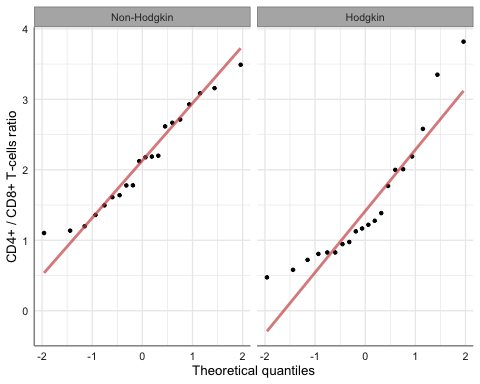Let’s say we choose a non-parametric test to compare the groups: wilcox.test(Ratio ~ Group, data = Hodgkin)  Wilcoxon rank sum exact test data: Ratio by Group W = 298, p-value = 0.007331 alternative hypothesis: true location shift is not equal to 0 ## 2.2 Graphical output For relatively small samples (for example, less than 30 observations per group) is a standard practice to show the actual data in dot plots with error bars. The pubh package offers two options to show graphically differences in continuous outcomes among groups: • For small samples: strip_error • For medium to large samples: bar_error For our current example: Hodgkin %>% strip_error(Ratio ~ Group)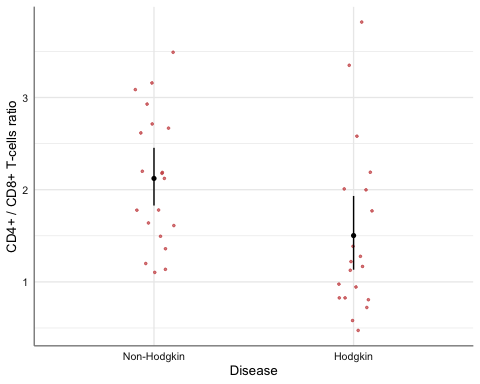The error bars represent 95% confidence intervals around mean values. Is relatively easy to add a line on top, to show that the two groups are significantly different. The function gf_star needs the reference point on how to draw an horizontal line to display statistical difference or to annotate a plot; in summary, gf_star: • Draws an horizontal line from $$(x1, y2)$$ to $$(x2, y2)$$. • Draws a vertical line from $$(x1, y1)$$ to $$(x1, y1)$$. • Draws a vertical line from $$(x2, y1)$$ to $$(x2, y1)$$. • Writes a character (the legend or “star”) at the mid point between $$x1$$ and $$x2$$ at high $$y3$$. Thus: $y1 < y2 < y3$ In our current example: Hodgkin %>% strip_error(Ratio ~ Group) %>% gf_star(x1 = 1, y1 = 4, x2 = 2, y2 = 4.05, y3 = 4.1, "**")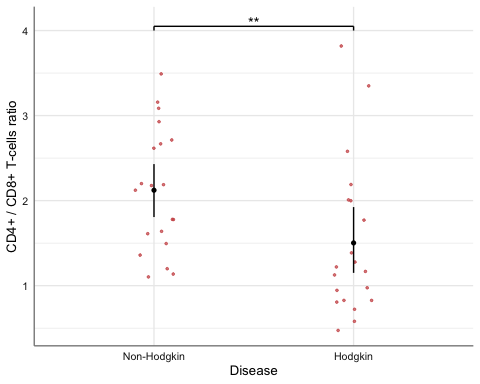For larger samples we could use bar charts with error bars. For example: data(birthwt, package = "MASS") birthwt <- birthwt %>% mutate( smoke = factor(smoke, labels = c("Non-smoker", "Smoker")), Race = factor(race > 1, labels = c("White", "Non-white")), race = factor(race, labels = c("White", "Afican American", "Other")) ) %>% var_labels( bwt = 'Birth weight (g)', smoke = 'Smoking status', race = 'Race', ) birthwt %>% bar_error(bwt ~ smoke)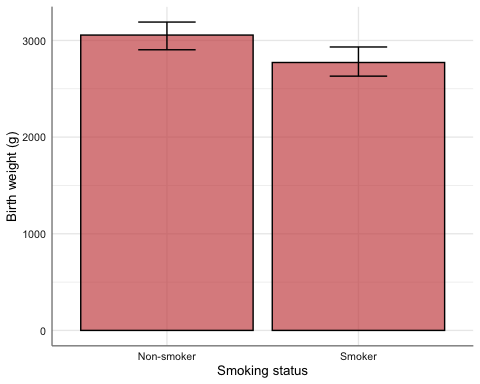Quick normality check: birthwt %>% qq_plot(~ bwt|smoke)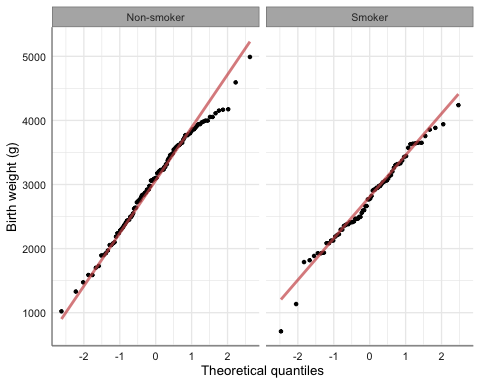The (unadjusted) $$t$$-test: birthwt %>% t_test(bwt ~ smoke, detailed = TRUE) %>% as.data.frame()  estimate estimate1 estimate2 .y. group1 group2 n1 n2 statistic p 1 283.7767 3055.696 2771.919 bwt Non-smoker Smoker 115 74 2.729886 0.007 df conf.low conf.high method alternative 1 170.1002 78.57486 488.9786 T-test two.sided The final plot with annotation to highlight statistical difference (unadjusted): birthwt %>% bar_error(bwt ~ smoke) %>% gf_star(x1 = 1, x2 = 2, y1 = 3400, y2 = 3500, y3 = 3550, "**")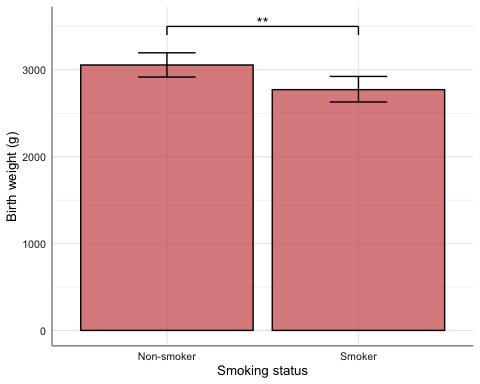Both strip_error and bar_error can generate plots stratified by a third variable, for example: birthwt %>% bar_error(bwt ~ smoke, fill = ~ Race) %>% gf_refine(ggsci::scale_fill_jama())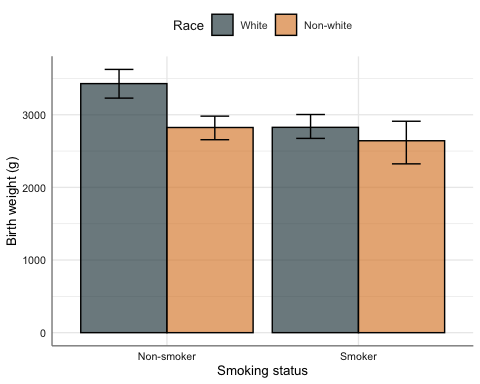birthwt %>% bar_error(bwt ~ smoke|Race)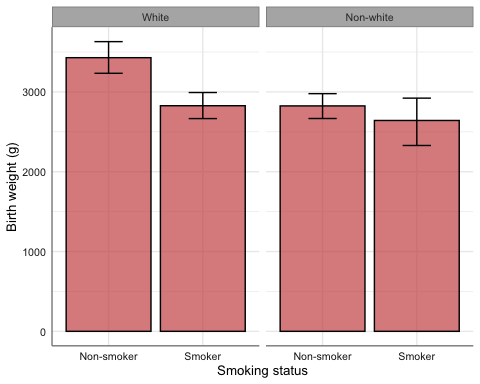birthwt %>% strip_error(bwt ~ smoke, pch = ~ Race, col = ~ Race) %>% gf_refine(ggsci::scale_color_jama())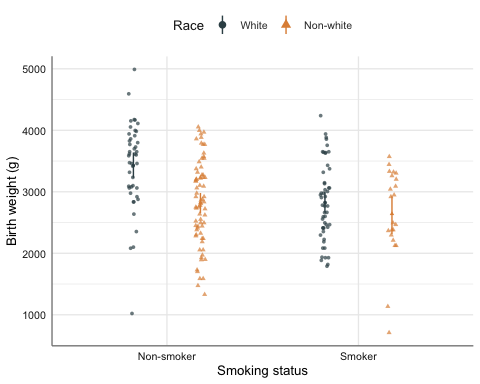# 3 Regression models The pubh package includes the function cosm_reg, which adds some cosmetics to objects generated by tbl_regression and huxtable. The function multiple helps in the analysis and visualisation of multiple comparisons. For simplicity, here we show the analysis of the linear model of smoking on birth weight, adjusting by race (and not by other potential confounders). model_bwt <- lm(bwt ~ smoke + race, data = birthwt) model_bwt %>% tbl_regression() %>% cosm_reg() %>% theme_pubh() %>% add_footnote(get_r2(model_bwt), font_size = 9)  Variable Beta 95% CI p-value Smoking status <0.001 Non-smoker — — Smoker -429 -644, -214 Race <0.001 White — — Afican American -450 -752, -148 Other -453 -683, -223 R2 = 0.123 Similar results can be obtained with the function glm_coef. model_bwt %>% glm_coef(labels = model_labels(model_bwt)) %>% as_hux() %>% theme_pubh() %>% set_align(everywhere, 2:3, "right") %>% add_footnote(get_r2(model_bwt), font_size = 9) ParameterCoefficientPr(>|t|) Constant3334.95 (3153.89, 3516.01)< 0.001 Smoking status: Smoker-428.73 (-643.86, -213.6)< 0.001 Race: Afican American-450.36 (-752.45, -148.27)0.004 Race: Other-452.88 (-682.67, -223.08)< 0.001 R2 = 0.123 In the last table of coefficients confidence intervals and p-values for levels of race are not adjusted for multiple comparisons. We can use functions from the emmeans package to make the corrections. multiple(model_bwt, ~ race)df
                 contrast estimate     SE t.ratio p.value lower.CL upper.CL
1 Afican American - White  -450.36 153.12   -2.94    0.01  -810.78   -89.94
2           Other - White  -452.88 116.48   -3.89 < 0.001  -727.04  -178.71
3 Other - Afican American    -2.52 160.59   -0.02       1  -380.53   375.49
multiple(model_bwt, ~ race)$fig_ci %>% gf_labs(x = "Difference in birth weights (g)")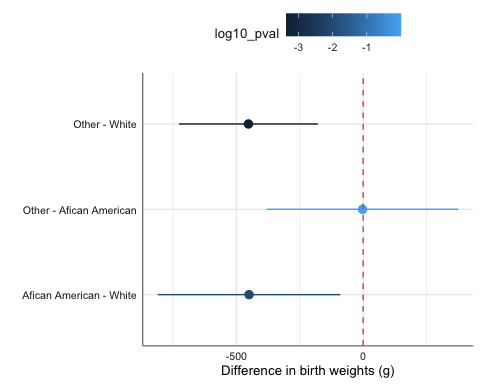multiple(model_bwt, ~ race)$fig_pval %>%
gf_labs(y = " ")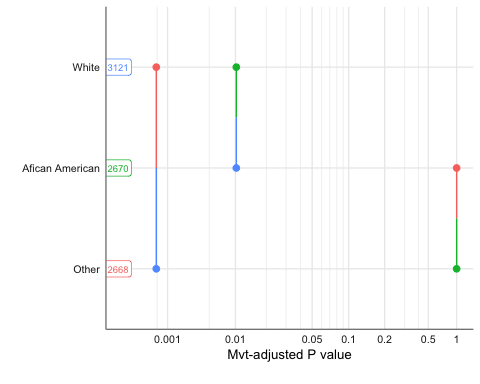# 4 Epidemiology functions

The pubh package offers two wrappers to epiR functions.

1. contingency calls epi.2by2 and it’s used to analyse two by two contingency tables.
2. diag_test calls epi.tests to compute statistics related with screening tests.

## 4.1 Contingency tables

Let’s say we want to look at the effect of ibuprofen on preventing death in patients with sepsis.

data(Bernard)
Bernard %>% head()
# A tibble: 6 × 9
id treat     race             fate  apache o2del followup temp0 temp10
<dbl> <fct>     <fct>            <fct>  <dbl> <dbl>    <dbl> <dbl>  <dbl>
1     1 Placebo   White            Dead      27  539.       50  35.2   36.6
2     2 Ibuprofen African American Alive     14   NA       720  38.7   37.6
3     3 Placebo   African American Dead      33  551.       33  38.3   NA
4     4 Ibuprofen White            Alive      3 1376.      720  38.3   36.4
5     5 Placebo   White            Alive      5   NA       720  38.6   37.6
6     6 Ibuprofen White            Alive     13 1523.      720  38.2   38.2

Let’s look at the table:

Bernard %>%
mutate(
fate = relevel(fate, ref = "Dead"),
treat = relevel(treat, ref = "Ibuprofen")
) %>%
copy_labels(Bernard) %>%
select(fate, treat) %>%
cross_tbl(by = "treat") %>%
theme_pubh(2)
 Treatment Ibuprofen, N = 224 Placebo, N = 231 Overall, N = 455 Mortality status Dead 84 (38%) 92 (40%) 176 (39%) Alive 140 (62%) 139 (60%) 279 (61%)

For epi.2by2 we need to provide the table of counts in the correct order, so we would type something like:

dat <- matrix(c(84, 140 , 92, 139), nrow = 2, byrow = TRUE)
epiR::epi.2by2(as.table(dat))
             Outcome +    Outcome -      Total        Inc risk *        Odds
Exposed +           84          140        224              37.5       0.600
Exposed -           92          139        231              39.8       0.662
Total              176          279        455              38.7       0.631

Point estimates and 95% CIs:
-------------------------------------------------------------------
Inc risk ratio                                 0.94 (0.75, 1.19)
Odds ratio                                     0.91 (0.62, 1.32)
Attrib risk in the exposed *                   -2.33 (-11.27, 6.62)
Attrib fraction in the exposed (%)            -6.20 (-33.90, 15.76)
Attrib risk in the population *                -1.15 (-8.88, 6.59)
Attrib fraction in the population (%)         -2.96 (-15.01, 7.82)
-------------------------------------------------------------------
Uncorrected chi2 test that OR = 1: chi2(1) = 0.260 Pr>chi2 = 0.610
Fisher exact test that OR = 1: Pr>chi2 = 0.631
Wald confidence limits
CI: confidence interval
* Outcomes per 100 population units 

For contingency we only need to provide the information in a formula:

Bernard %>%
contingency(fate ~ treat) 
           Outcome
Ibuprofen   84   140
Placebo     92   139

Outcome +    Outcome -      Total        Inc risk *        Odds
Exposed +           84          140        224              37.5       0.600
Exposed -           92          139        231              39.8       0.662
Total              176          279        455              38.7       0.631

Point estimates and 95% CIs:
-------------------------------------------------------------------
Inc risk ratio                                 0.94 (0.75, 1.19)
Odds ratio                                     0.91 (0.62, 1.32)
Attrib risk in the exposed *                   -2.33 (-11.27, 6.62)
Attrib fraction in the exposed (%)            -6.20 (-33.90, 15.76)
Attrib risk in the population *                -1.15 (-8.88, 6.59)
Attrib fraction in the population (%)         -2.96 (-15.01, 7.82)
-------------------------------------------------------------------
Uncorrected chi2 test that OR = 1: chi2(1) = 0.260 Pr>chi2 = 0.610
Fisher exact test that OR = 1: Pr>chi2 = 0.631
Wald confidence limits
CI: confidence interval
* Outcomes per 100 population units

Pearson's Chi-squared test with Yates' continuity correction

data:  dat
X-squared = 0.17076, df = 1, p-value = 0.6794

Advantages of contingency:

1. Easier input without the need to create the table.
2. Displays the standard epidemiological table at the start of the output. This aids to check what are the reference levels on each category.
3. In the case that the $$\chi^2$$-test is not appropriate, contingency would show the results of the Fisher exact test at the end of the output.

### 4.1.1 Mantel-Haenszel odds ratio

For mhor the formula has the following syntax:

outcome ~ stratum/exposure, data = my_data

Thus, mhor displays the odds ratio of exposure yes against exposure no on outcome yes for different levels of stratum, as well as the Mantel-Haenszel pooled odds ratio.

Example: effect of eating chocolate ice cream on being ill by sex from the oswego data set.

data(oswego, package = "epitools")

oswego <- oswego %>%
mutate(
ill = factor(ill, labels = c("No", "Yes")),
sex = factor(sex, labels = c("Female", "Male")),
chocolate.ice.cream = factor(chocolate.ice.cream, labels = c("No", "Yes"))
) %>%
var_labels(
ill = "Developed illness",
sex = "Sex",
chocolate.ice.cream = "Consumed chocolate ice cream"
)
oswego %>%
mhor(ill ~ sex/chocolate.ice.cream)

OR Lower.CI Upper.CI Pr(>|z|)
sexFemale:chocolate.ice.creamYes 0.47     0.11     2.06    0.318
sexMale:chocolate.ice.creamYes   0.24     0.05     1.13    0.072

Common OR Lower CI Upper CI Pr(>|z|)
Cochran-Mantel-Haenszel:       0.35     0.12     1.01    0.085

Test for effect modification (interaction): p =  0.5434


## 4.2 Diagnostic tests

Example: We compare the use of lung’s X-rays on the screening of TB against the gold standard test.

Freq <- c(1739, 8, 51, 22)
BCG <- gl(2, 1, 4, labels=c("Negative", "Positive"))
Xray <- gl(2, 2, labels=c("Negative", "Positive"))
tb <- data.frame(Freq, BCG, Xray)
tb <- expand_df(tb)

diag_test(BCG ~ Xray, data = tb)
          Outcome +    Outcome -      Total
Test +           22           51         73
Test -            8         1739       1747
Total            30         1790       1820

Point estimates and 95% CIs:
--------------------------------------------------------------
Apparent prevalence *                  0.04 (0.03, 0.05)
True prevalence *                      0.02 (0.01, 0.02)
Sensitivity *                          0.73 (0.54, 0.88)
Specificity *                          0.97 (0.96, 0.98)
Positive predictive value *            0.30 (0.20, 0.42)
Negative predictive value *            1.00 (0.99, 1.00)
Positive likelihood ratio              25.74 (18.21, 36.38)
Negative likelihood ratio              0.27 (0.15, 0.50)
False T+ proportion for true D- *      0.03 (0.02, 0.04)
False T- proportion for true D+ *      0.27 (0.12, 0.46)
False T+ proportion for T+ *           0.70 (0.58, 0.80)
False T- proportion for T- *           0.00 (0.00, 0.01)
Correctly classified proportion *      0.97 (0.96, 0.98)
--------------------------------------------------------------
* Exact CIs

Using the immediate version (direct input):

diag_test2(22, 51, 8, 1739)

Outcome +    Outcome -      Total
Test +           22           51         73
Test -            8         1739       1747
Total            30         1790       1820

Point estimates and 95% CIs:
--------------------------------------------------------------
Apparent prevalence *                  0.04 (0.03, 0.05)
True prevalence *                      0.02 (0.01, 0.02)
Sensitivity *                          0.73 (0.54, 0.88)
Specificity *                          0.97 (0.96, 0.98)
Positive predictive value *            0.30 (0.20, 0.42)
Negative predictive value *            1.00 (0.99, 1.00)
Positive likelihood ratio              25.74 (18.21, 36.38)
Negative likelihood ratio              0.27 (0.15, 0.50)
False T+ proportion for true D- *      0.03 (0.02, 0.04)
False T- proportion for true D+ *      0.27 (0.12, 0.46)
False T+ proportion for T+ *           0.70 (0.58, 0.80)
False T- proportion for T- *           0.00 (0.00, 0.01)
Correctly classified proportion *      0.97 (0.96, 0.98)
--------------------------------------------------------------
* Exact CIs